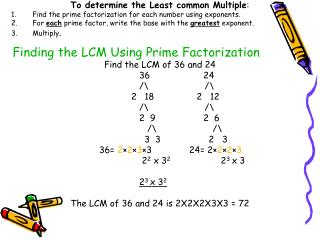DownloadDownload PresentationFinding the LCM Using Prime Factorization

# Finding the LCM Using Prime Factorization

Download Presentation## Finding the LCM Using Prime Factorization

- - - - - - - - - - - - - - - - - - - - - - - - - - - E N D - - - - - - - - - - - - - - - - - - - - - - - - - - -
##### Presentation Transcript

1. Finding the LCM Using Prime Factorization To determine the Least common Multiple: • Find the prime factorization for each number using exponents. • For each prime factor, write the base with the greatest exponent. • Multiply. Find the LCM of 36 and 24 36 24 /\ /\ 2 18 2 12 /\ /\ 2 9 2 6 /\ /\ 3 3 2 3 36= 2×2×3×3 24= 2×2×2×3 22 x 32 23 x 3 23 x 32 The LCM of 36 and 24 is 2X2X2X3X3 = 72

2. FINDING THE LCM USING PRIME FACTORIZATION • Prime factor each number. Write using exponents. • For each prime factor, write the base with the greatest • exponent. • 3. Multiply all together. Find the GCF of 36 and 24 36 24 /\ /\ 2 18 2 12 /\ /\ 2 9 2 6 /\ /\ 3 3 2 3 36= 2×2×3×3 24= 2×2×2×3 22 x 32 23 x 3 22 x 3 The GCF of 36 and 24 is 2×2×3=12